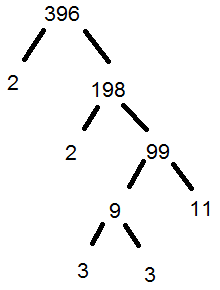# In math tutoring, prime numbers and prime factorization are receiving fresh attention.  We’ll briefly look at the concepts.

Before we talk about prime numbers, let’s define factor.  In math, a factor is a number that divides into another number with no remainder.  Therefore, 4 is a factor of 12; 7 is a factor of 14.

We also need to define product.  A product is the answer to a multiplication.  Therefore, the product of 2 and 5 is 10.  We could also say that 36 is the product of 9 and 4.

A prime number is a number with only two factors:  itself and one.  The number 1 is not prime, since it only has one factor:  1.  However, 2 is prime, as are 3, 5, 7,11,13, 19, and so on.  Note that 9 is not prime, since 9 has 3 as a factor besides itself and 1.

The prime factorization of a number is the number stated as a product of primes.  Each number has a unique prime factorization, if you discount the different orders.  The prime factorization of 10 is 2×5.  The prime factorization of 48 is 2x2x2x2x3, or 24x3.  Note, once again, that the prime factorization only includes prime numbers as defined above.

To illustrate how to get the prime factorization of a number, you might use the ancient “factor tree” method.  For example, imagine you need the prime factorization of 396:Notice each path ends with a prime number.  Collecting them all gives 396=2x2x3x3x11, or 396=2²x3²x11.

We’ll explore some uses of the prime factorization in future posts.

Jack of  Oracle Tutoring by Jack and Diane, Campbell River, BC